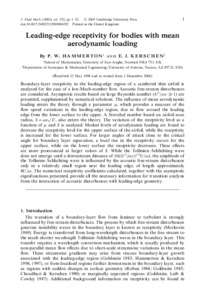Hammerton, PW and Kerschen, E.J (2005) Leading-edge receptivity for bodies with mean aerodynamic loading. Journal of Fluid Mechanics, 535. pp. 1-32. ISSN 0022-1120Preview
PDF (JFM05.pdf)
Boundary-layer receptivity in the leading-edge region of a cambered thin airfoil is analysed for the case of a low-Mach-number flow. Acoustic free-stream disturbances are considered. Asymptotic results based on large Reynolds number ($U^2 / \omega \nu \,{\gg}\, 1$) are presented, supplemented by numerical solutions. The influence of mean aerodynamic loading enters the theory through a parameter $\mu$, which provides a measure of the flow speed variations in the leading-edge region, due to flow around the leading edge from the lower surface to the upper. A Strouhal number based on airfoil nose radius, $S\,{=}\,\omega r_n/U$, also enters the theory. The variation of the receptivity level as a function of $\mu$ and $S$ is analysed. Modest levels of aerodynamic loading are found to decrease the receptivity level for the upper surface of the airfoil, while the receptivity is increased for the lower surface. For larger angles of attack close to the critical angle for boundary layer separation, a local rise in the receptivity occurs for the upper surface, while on the lower surface the receptivity decreases. These effects are more pronounced at larger values of $S$. While the Tollmien–Schlichting wave does not emerge until a downstream distance of $O((U^2 / \omega \nu)^{1/3} U / \omega)$, the amplitude of the Tollmien–Schlichting wave is influenced by the acoustic free-stream disturbances only in a relatively small region near the leading edge, of length approximately $4 U/\omega$. The numerical receptivity coefficients calculated, together with the asymptotic eigenfunctions presented, provide all the necessary information for transition analysis from the interaction of acoustic disturbances with leading-edge geometry.View Item